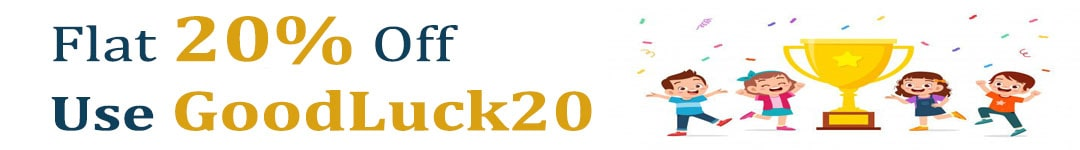International Maths Olympiad Forum By SOF Olympiad Trainer - Page 580

# User ForumSubject :IMO    Class : Class 5

## Ans 1:

Class : Class 9
The ans is C

Subject :IMO    Class : Class 6

## Ans 1:

Class : Class 6
The formula is (L.C.M.*H .C.F.)/1st number.

Subject :IMO    Class : Class 4

## Ans 1:

Class : Class 4
ans a-80. From 1-9 there are 9 digits . so we are left with 151-9=142 digits. Note that from 10 onwards all numbers are of 2 digits. So there are 142/2=71 numbers to have 142 digits. Therefore there are 9+71=80 numbers.

Subject :IMO    Class : Class 4

## Ans 1:

Class : Class 4
Youâre are supposed to multiply the number by 3 hence the answer is C

## Ans 2:

Class : Class 6
Answer should only and only be D

## Ans 3:

Class : Class 7
The no is 31.31x5=155 209=364.Hence neither statement 1 nor statement 2 is correct

Class : Class 4

## Ans 5:

Class : Class 6
(364-209) divided by 5=3131*3=93So, the answer is C.

Class : Class 4
ans-d

Class : Class 4

## Ans 8:

Class : Class 6

Subject :IMO    Class : Class 5

Class : Class 5
C

Class : Class 9
The ans is C 48

## Ans 3:

Class : Class 7
The answer is c

Subject :IMO    Class : Class 5

## Ans 1: (Master Answer)

Class : Class 1
The correct answer is A.

Subject :IMO    Class : Class 3

## Ans 1:

Class : Class 4
the shapes are not clear

Subject :IMO    Class : Class 5

#### View All Answers (0)

Subject :IMO    Class : Class 5

Class : Class 9
ans =1036844

Class : Class 9
ans =1036844